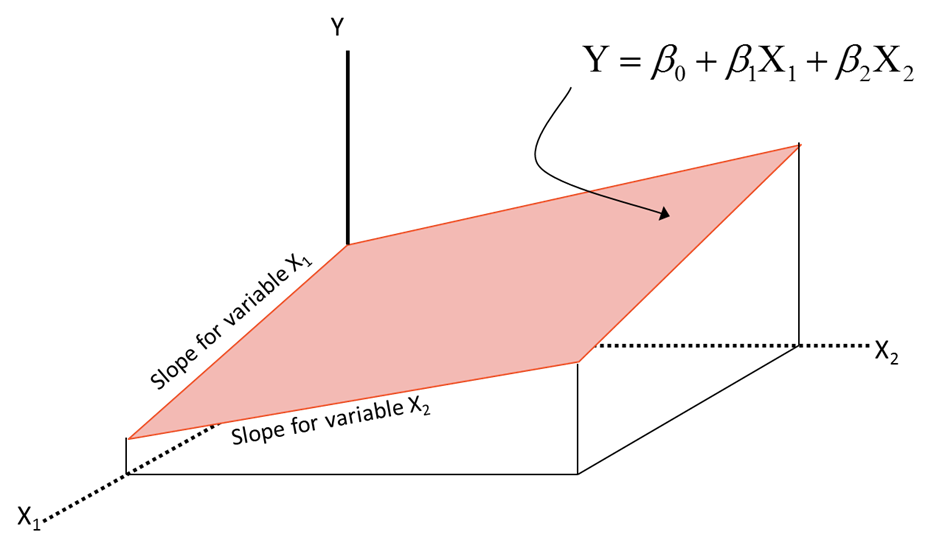• No products in the cart.

# 204.1.6 Multiple Regression in Python

##### Dealing with more than one input variable in Linear Regression.

Link to the previous post: https://statinfer.com/204-1-5-r-squared-in-python/

For the last few posts of the machine learning blog series 204, we were just going through single input variable regression. In this post, we will see how to take care of multiple input variables.

## Multiple Regression

• Using multiple predictor variables instead of single variable
• We need to find a perfect plane here### Code – Multiple Regression

In :
from sklearn.linear_model import LinearRegression
lr = LinearRegression()
lr.fit(air[["Promotion_Budget"]+["Inter_metro_flight_ratio"]], air[["Passengers"]])

Out:
LinearRegression(copy_X=True, fit_intercept=True, n_jobs=1, normalize=False)
In :
predictions = lr.predict(air[["Promotion_Budget"]+["Inter_metro_flight_ratio"]])

In :
import statsmodels.formula.api as sm
model = sm.ols(formula='Passengers ~ Promotion_Budget+Inter_metro_flight_ratio', data=air)
fitted = model.fit()
fitted.summary()

Out:
Dep. Variable: R-squared: Passengers 0.934 OLS 0.932 Least Squares 540.5 Wed, 27 Jul 2016 4.76e-46 11:48:27 -750.96 80 1508. 77 1515. 2 nonrobust
coef std err t P>|t| [95.0% Conf. Int.] 2017.7724 1624.803 1.242 0.218 -1217.624 5253.169 0.0707 0.002 28.297 0.000 0.066 0.076 -2121.5208 2473.189 -0.858 0.394 -7046.268 2803.227
 Omnibus: Durbin-Watson: 26.259 1.8 0 5.075 -0.096 0.0791 1.781 5.25e+06

### Individual Impact of variables

• Look at the P-value
• Probability of the hypothesis being right.
• Individual variable coefficient is tested for significance
• Beta coefficients follow t distribution.
• Individual P values tell us about the significance of each variable
• A variable is significant if P value is less than 5%. Lesser the P-value, better the variable
• Note it is possible all the variables in a regression to produce a great fit, and yet very few of the variables be individually significant.

To test

H0:βi=0
Ha:βi0

Test Statistic

t=bi/s(bi)

Reject H0 if

t>t(α2;nk1)

or

t>t(α2;nk1)

### Practice : Multiple Regression

• Build a multiple regression model to predict the number of passengers
• What is R-square value
• Are there any predictor variables that are not impacting the dependent variable
In :
#Build a multiple regression model to predict the number of passengers

from sklearn.linear_model import LinearRegression
lr = LinearRegression()
lr.fit(air[["Promotion_Budget"]+["Inter_metro_flight_ratio"]+["Service_Quality_Score"]], air[["Passengers"]])
predictions = lr.predict(air[["Promotion_Budget"]+["Inter_metro_flight_ratio"]+["Service_Quality_Score"]])

In :
import statsmodels.formula.api as sm
model = sm.ols(formula='Passengers ~ Promotion_Budget+Inter_metro_flight_ratio+Service_Quality_Score', data=air)
fitted = model.fit()
fitted.summary()

Out:
Dep. Variable: R-squared: Passengers 0.951 OLS 0.949 Least Squares 495.6 Wed, 27 Jul 2016 8.71e-50 11:48:28 -738.45 80 1485. 76 1494. 3 nonrobust
coef std err t P>|t| [95.0% Conf. Int.] 1.921e+04 3542.694 5.424 0.000 1.22e+04 2.63e+04 0.0555 0.004 15.476 0.000 0.048 0.063 -2003.4508 2129.095 -0.941 0.350 -6243.912 2237.010 -2802.0708 530.382 -5.283 0.000 -3858.419 -1745.723
 Omnibus: Durbin-Watson: 6.902 2.312 0.032 2.759 -0.051 0.252 2.096 8.22e+06
• What is R-square value

0.951

• Are there any predictor variables that are not impacting the dependent variable

Inter_metro_flight_ratio